WPS Office

Free All-in-One Office Suite with PDF Editor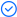Read, edit, and convert PDFs with the powerful PDF toolkit.Microsoft-like interface, easy to use.

Windows • MacOS • Linux • iOS • Android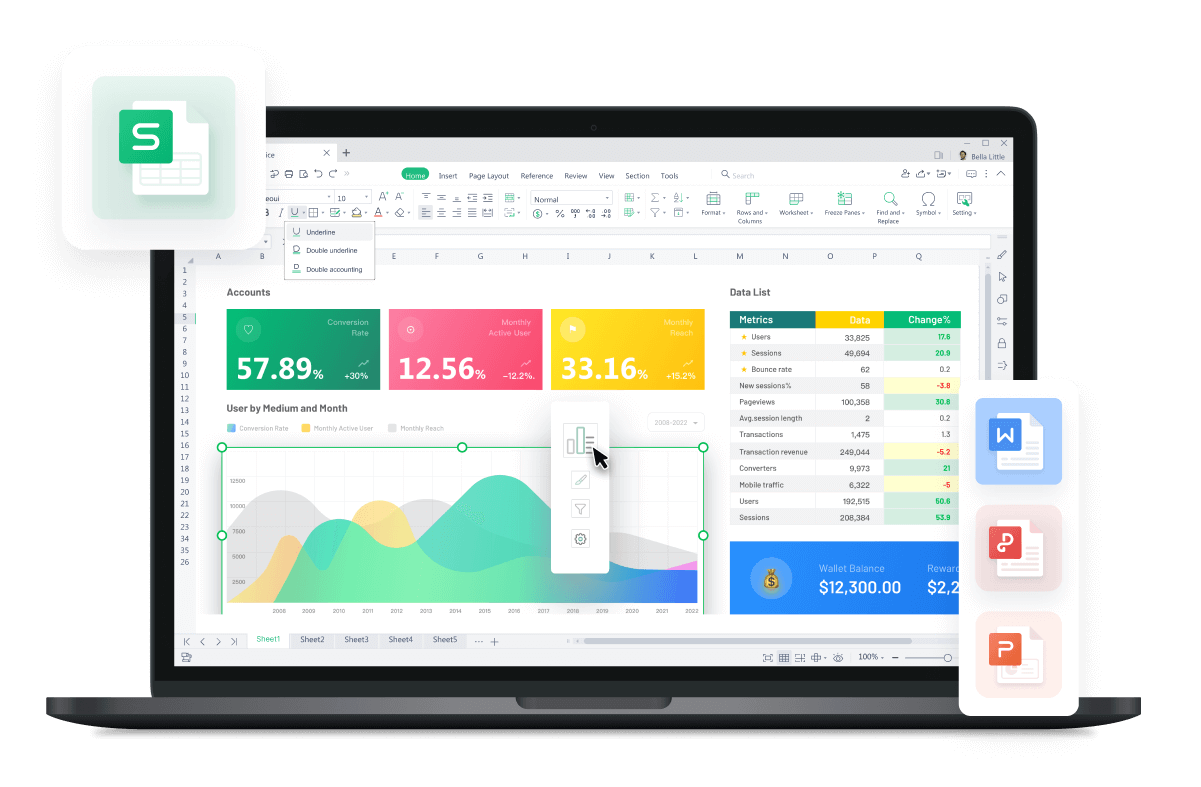# How to Multiply Two Cells in Excel Spreadsheet Using WPS Office:A Step-by-Step Guide

November 20, 2023
140 Views

The multiplication function is integral to various aspects of our lives. It's just as essential as addition or subtraction, and some argue it's even more important. While addition and subtraction can be easily performed, dealing with larger numbers and complex situations, such as financial analysis or budgeting, often requires accurate multiplication. In this article, we'll guide you through how to multiply two cells in excel spreadsheet, which can come in handy and prove to be invaluable in the long run.

## Why You Should Apply Multiplication Formulas to Excel Spreadsheets

Utilizing the multiplication features in Microsoft Excel is advantageous for various tasks. These features streamline the computation of multiple data sets, facilitating the calculation of product sums and multiplication across a broader range of numbers. For instance, when dealing with extensive datasets, Excel provides diverse commands that simplify intricate multiplication operations.

Moreover, the application of summation notations within Excel allows for the seamless combination of multiplication and addition. The multiplication features in Excel are instrumental in simplifying formulas that involve multiple operations, contributing to enhanced efficiency in handling numerical data.

## Easiest Way to Multiply Two Cells in Excel Using WPS Spreadsheet

### Using Asterisk Symbol (*)

Learn how to multiply two cells in Excel using WPS Spreadsheet with the Asterisk symbol. This is the simplest method for multiplying cells in WPS Spreadsheet, let's illustrate by multiplying cells A2 and B3.

Step 1: Open WPS Spreadsheet, click on an empty cell to apply the function.

Step 2: Begin the function by entering the "=" symbol and select the first cell (A2).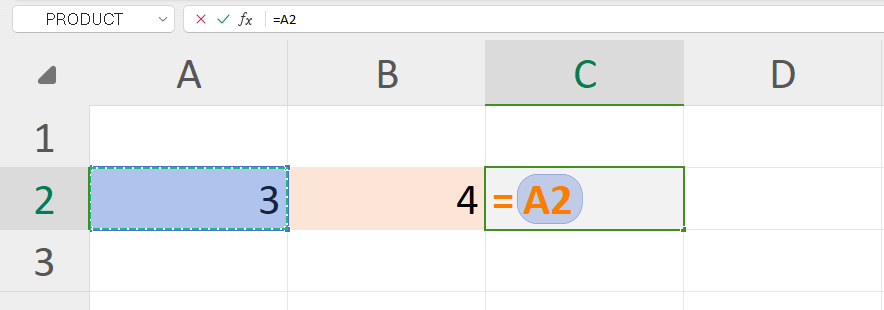Step 3: Enter the Asterisk Symbol "*", followed by selecting the next cell (B2).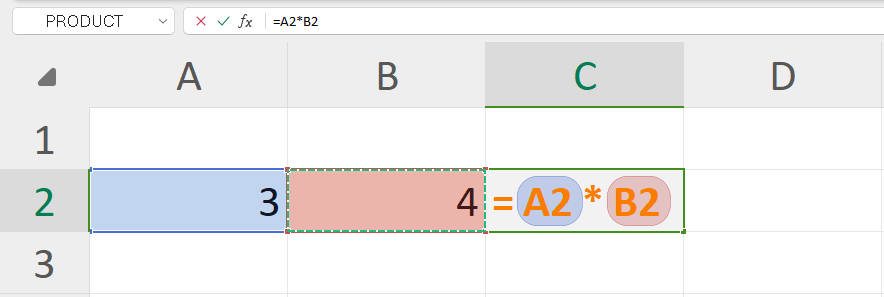Step 4: Press “Enter” to obtain your multiplication results in WPS Spreadsheet.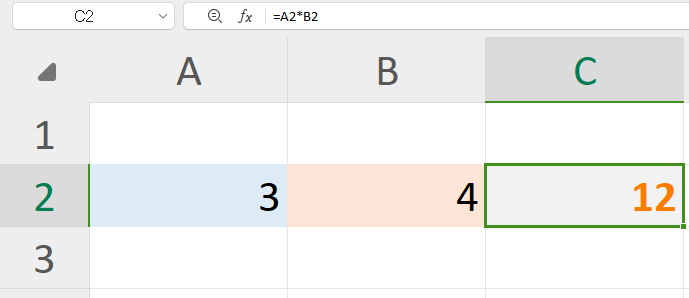## How to Multiply a Range of Cells in Excel Spreadsheet

### Using the PRODUCT Function in WPS Spreadsheet

In this section, let's learn how to multiply a range of cells in Excel spreadsheet using WPS Office. Let's demonstrate with a simple example using cells A3, B3, and C3.

Step 1: Click on an empty cell where the PRODUCT function will be applied. Enter the “Equal sign” to initiate the function.

Step 2: Type "PRODUCT" after the equal sign and press the "Tab" key to select the PRODUCT function.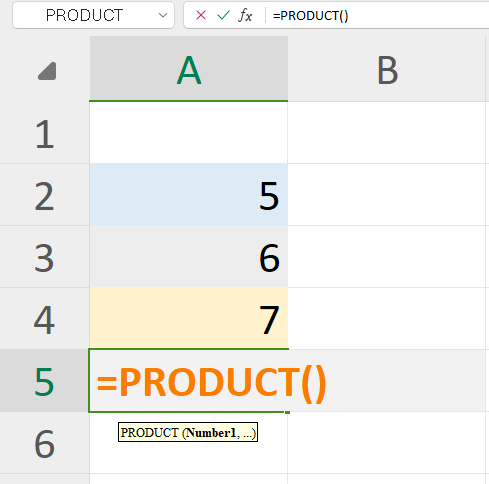Step 3: Place the cursor on cell A3, click, and drag to cell C3 to select the cell range.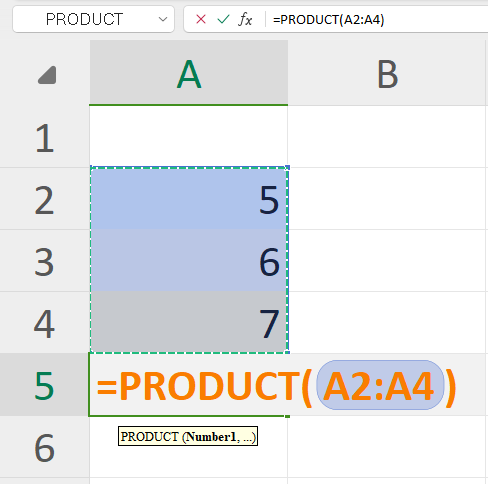Note: WPS Office automatically inserts closing parentheses, reducing the chance of human errors.

Step 4: Press “Enter” on your keyboard, and the results will be displayed on your WPS Spreadsheet.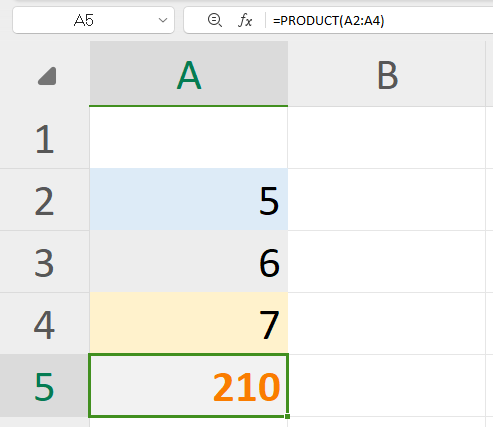Note: Our functions are versatile, applicable to both rows and columns for increased flexibility in data manipulation.## How to Multiply Arrays in Excel Spreadsheet

### Using the SUMPRODUCT Function in WPS Spreadsheet

Another essential function leveraging the product function is the SUMPRODUCT function in WPS Spreadsheet. Here’s an example for better understanding:

Let's consider a scenario where we have sales data for three products in a regular store. Now, we want to calculate the total sales for this store within a particular day.

To achieve this, we need to individually multiply the quantity sold with the price for each product and then sum up these products. This will give us the total sales of this store.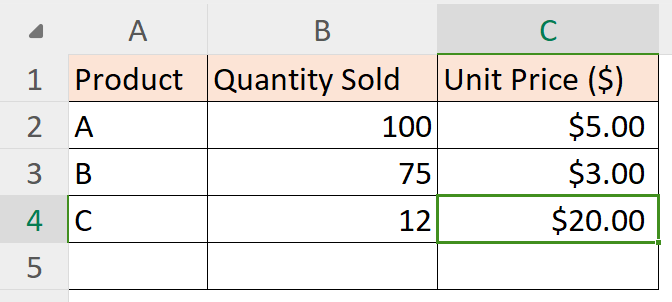Step 1: With the sales spreadsheet open in Excel, click on an empty cell to run the SUMPRODUCT function.

Step 2: Start the function by entering the "Equals sign" symbol and then input the SUMPRODUCT function.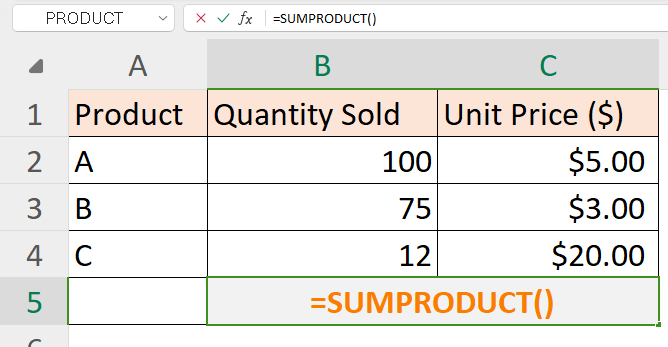Step 3: Choose the first range of cells; in our example, we'll use the quantity sold as our first range of cells.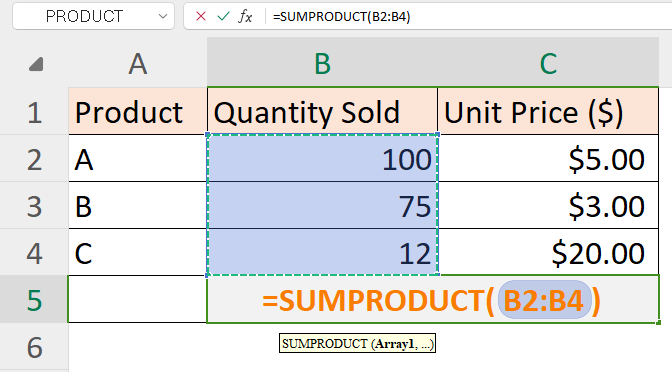Step 4: For the second range of cells, we have the unit price of each product.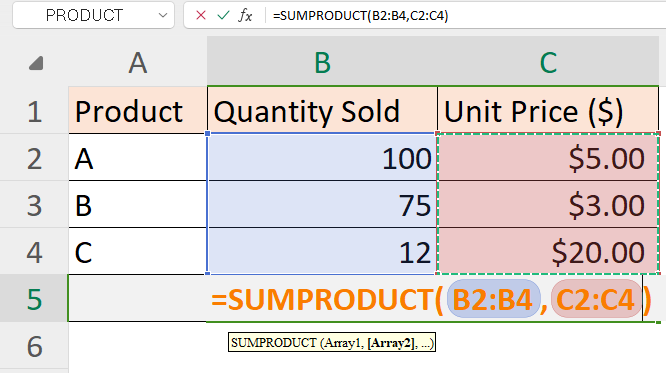Step 5: Press “Enter” to allow WPS Spreadsheet to calculate the result of the SUMPRODUCT function.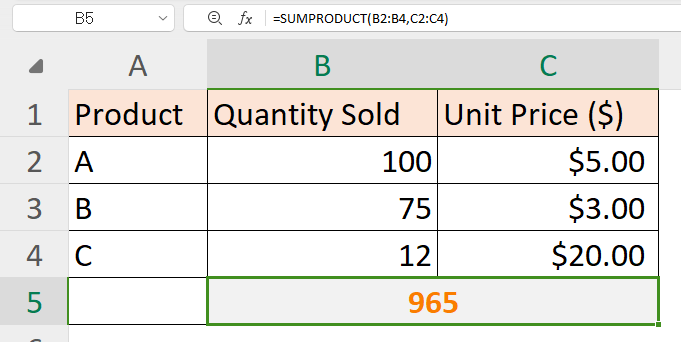We hope this step-by-step guide on how to use multiplication formulas in a spreadsheet using WPS Office Proves valuable to you and your analytical endeavors.

## Tips and Tricks

Seeking to make multiplication more engaging and user-friendly? Here are some tips and tricks for WPS Office users.

• Error-Reduction Power: Leverage WPS Office's automatic closing parentheses feature to minimize errors, especially in complex multiplication formulas.

• Customized Efficiency: Enhance your workflow by personalizing WPS Office settings and toolbar, ensuring swift access to frequently used multiplication functions.

• Continuous Learning: Unlock the full potential of WPS Office by expanding your knowledge beyond basic multiplication, exploring and mastering advanced functions for seamless spreadsheet proficiency.

Trustpilot4.8
WPS Office- Free All-in-One Office Suite

• Edit PDF files with the powerful PDF toolkit.

• Microsoft-like interface. Easy to learn. 100% Compatibility.

• Boost your productivity with WPS's abundant free Word, Excel, PPT, and CV templates.

5,820,008 UserAlgirdas Jasaitis
logo

## FAQs

### Q1. How to do calculations in Excel Spreadsheet?

Step 1: Begin with the equal symbol (=) to indicate a formula.

Step 2: Enter the desired equation; for instance, =5+7 for addition.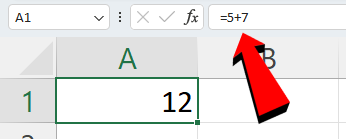Step 3: Press Enter to execute the calculation.

### Q2. How to change the order of calculations in Excel Spreadsheet?

Similar to math, Excel lets you adjust the calculation order with parentheses.

For example, in the calculation =3+5*2, Excel multiplies 5 by 2 first, then adds 3. The result is 13. By using parentheses like this: =3+(5*2), you instruct Excel to multiply 5 by 2 first, then add 3. The result is 13, maintaining the desired order.

### Q3. Can I apply multiplication formulas to Excel spreadsheets on mobile devices using WPS Office?

Yes, you can use WPS Office on your phone or tablet to multiply numbers in Excel. Applying multiplication formulas is straightforward, making it convenient for calculations anytime, anywhere. WPS Office is designed for mobile use, providing a seamless experience on your smartphone or tablet.

## Effortless Excel Multiplication with WPS Office

Efficient data handling becomes ten times easier when you know how to multiply two cells in excel spreadsheet, because then you have the multiplication formula at your fingertips. Once you're comfortable with this formula, you're well-equipped to execute a variety of calculations effortlessly.

WPS Office offers a comprehensive office suite, complete with a free spreadsheet tool that simplifies various functions. Download WPS Office today for a seamless and user-friendly experience.